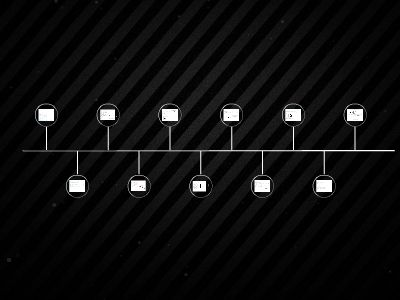Publications: 91 | Followers: 0

## www.math.drexel.edu

Publish on Category: Birds 0

Parametric Equations of lines
Math 200Week 2 - Wednesday
Math 200
Main Questions for Today
How do we describe lines in space?How do we determine if two lines areparallel,intersecting, orskew?How do we figure out where lines intersection the coordinate planes?
Math 200
Describing the points on a particular line
A line is uniquely defined bytwo points(just like in 2D)We can draw a bunch of vectors between these points and the originWe can use these vectors to come up with a set of equations for any point on the line…
P1(x1,y1,z1)
P2(x2,y2,z2)
<x1,y1,z1>
<x2,y2,z2>
<a, b, c>
We now know that this vector is <x2-x1, y2-y1, z2-z1>, but we’ll just write <a,b,c> for short
Math 200
We want to describe anarbitrarypoint on the line in terms of the vectors we drew<x1, y1, z1> will get us to P1<a, b, c> gets us from P1to P2Scalar multiplesof <a, b, c> will go back and forth along the line!
(x,y,z)
<x1,y1,z1>
<a, b, c>
<x,y,z>
t<a, b, c>
Math 200
What’s the relationship between the vector that points directly to <x,y,z> and the other vectors?<x,y,z> = <x1,y1,z1> + t<a,b,c><x,y,z> = <x1+at, y1+bt, z1+ct>
(x,y,z)
<x1,y1,z1>
<a, b, c>
<x,y,z>
t<a, b, c>
Math 200
Example 1
Compute a set of parametric equations for the line containing the points A(1,3,4) and B(-2,1,7)First, wefind a vector parallel to the linev= <-2 - 1, 1 - 3, 7 - 4> = <-3, -2, 3>Now we pick a starting pointEither A or B will do
Math 200
You try one
Find a set of parametric equations for the line containing the points A(4,-2,0) and B(3,3,-1).Find another point on the line.
To get another point on the line, we can just plug in another “time” value fort.E.g. Whent= -1 we get (5, -7, 1)
Math 200
Another Example
Find a set of parametric equations for the line that passes through the point A(1,4,2) and is parallel to thexy-plane and theyz-plane.If it’s parallel to both thexy-plane and theyz-plane, then its direction vector must havex-andz-components that are zeroe.g. <0,1,0> would work
4
Math 200
Parallel, Intersecting, or skew
ParallelParallel lines have parallel direction vectorse.g.
The first line is parallel to the vector <-2,1,1>The second line is parallel to <4,-2,-2>Since, -2<-2,1,1> = <4,-2-2>, the lines are parallel.
Math 200
Skew vs intersectingSuppose we have two lines that are not parallele.g.
We know they’re not parallel because <-1,3,-2> is not parallel to <4,-1,1>.Theymayintersect, but they may pass right by one another…
Even if they do intersect, they may pass through their common point for differentt-values!
Rather than setting the equations equal as given, we’ll needdifferent lettersfor the parameter in each!
Pick two equations and solve foraandb

0

Embed

Share